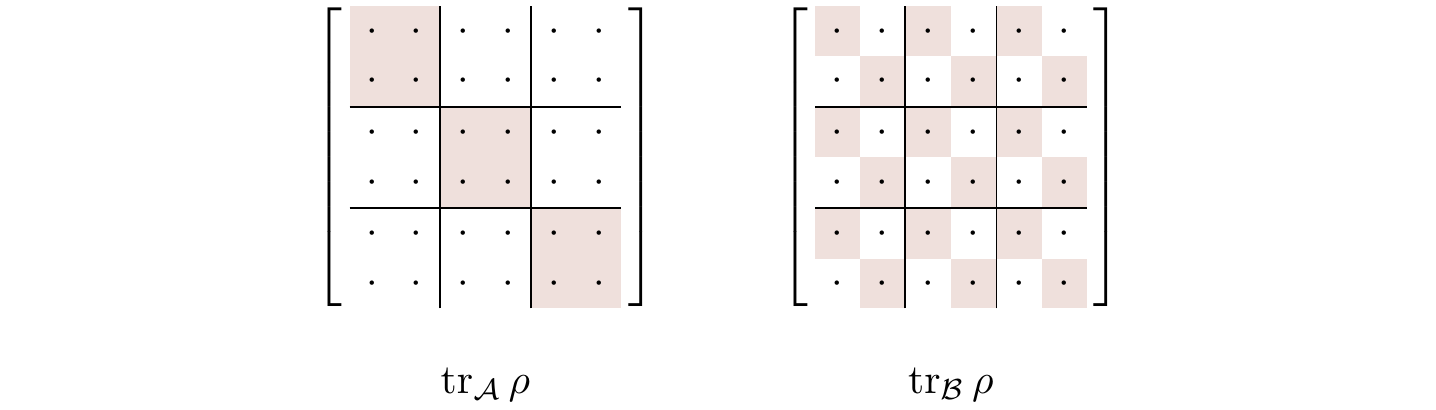## 6.7 Partial trace, revisited

You can calculate the trace of a matrix by summing its diagonal entries. Can you do something similar to calculate the partial trace of a density matrix? Suppose someone writes down for you a density matrix of two qubits in the standard basis, \{|00\rangle, |01\rangle, |10\rangle, |11\rangle\}, and asks you to find the reduced density matrices of the individual qubits. The tensor product structure of this (4\times 4) matrix means that it is has a block form: \rho_{\mathcal{AB}} = \left[ \begin{array}{c|c} P & Q \\\hline R & S \end{array} \right] where P,Q,R,S are (2\times 2) sized sub-matrices.

The two partial traces can then be evaluated as114 \begin{aligned} \rho_{\mathcal{A}} &= \operatorname{tr}_{\mathcal{B}}\rho_{\mathcal{AB}} = \left[ \begin{array}{c|c} \operatorname{tr}P & \operatorname{tr}Q \\\hline \operatorname{tr}R & \operatorname{tr}S \end{array} \right] \\\rho_{\mathcal{B}} &= \operatorname{tr}_{\mathcal{A}}\rho_{\mathcal{AB}} = P+S. \end{aligned}

In general, for any matrix \rho in \mathcal{H}_{\mathcal{A}}\otimes\mathcal{H}_{\mathcal{B}} that is written in the tensor product basis, the partial trace over \mathcal{A} is the sum of the diagonal block matrices, and the partial trace over \mathcal{B} is the matrix in which the block sub-matrices are replaced by their traces — see Figure 6.1.Figure 6.1: Visualising the two partial traces of a matrix written in the tensor product basis.

To better understand the partial trace, it helps to give a more abstract definition. It turns out that the partial trace over \mathcal{B} can be defined as the unique map \rho_{\mathcal{AB}}\mapsto\rho_{\mathcal{A}} such that115 \operatorname{tr}[X\rho_{\mathcal{A}}] = \operatorname{tr}[(X\otimes\mathbf{1})\rho_{\mathcal{AB}}] \tag{$\circledast$} holds for any observable X acting on \mathcal{A}, where \mathbf{1} is the identity operator acting on \mathcal{B}. This condition ensures the consistency of statistical predictions: any observable X on \mathcal{A} can be viewed as an observable X\otimes\mathbf{1} on the composite system \mathcal{AB}; when constructing \rho_{\mathcal{A}}, we had better make sure that for any observable X the average value of X in the state \rho_{\mathcal{A}} is the same as the average value of X\otimes\mathbf{1} in the state \rho_{\mathcal{AB}}. This is exactly what the condition in (\circledast) guarantees.

To show that our more ad-hoc definition of the partial trace agrees with this slightly more abstract one, consider again some state |\psi_{\mathcal{AB}}\rangle written in the form \begin{aligned} |\psi_{\mathcal{AB}}\rangle &= \sum_{i,j} c_{ij}|a_i\rangle\otimes|b_j\rangle \\&= \sum_{j} |\widetilde\psi_j\rangle|b_j\rangle = \sum_{j} \sqrt{p_j}|\psi_j\rangle|b_j\rangle. \end{aligned} Now assume that Alice measures some observable X on her part of the system. Such an observable can be thought of as X\otimes\mathbf{1}, acting on the entire system. The expected value of this observable in the state |\psi_{\mathcal{AB}}\rangle is, by definition, \operatorname{tr}(X\otimes\mathbf{1})|\psi_{\mathcal{AB}}\rangle\langle\psi_{\mathcal{AB}}|, and \begin{aligned} \operatorname{tr}[(X\otimes \mathbf{1}) \rho_{\mathcal{AB}}] &= \operatorname{tr}\left[ (X\otimes\mathbf{1}) \left( \sum_{i,j} |\widetilde\psi_i\rangle\langle\widetilde\psi_j| \otimes |b_i\rangle\langle b_j| \right) \right] \\&= \sum_{i,j} \left[ \operatorname{tr}\left(X |\widetilde\psi_i\rangle\langle\widetilde\psi_j|\right) \right] \underbrace{\left[\operatorname{tr}\left(|b_i\rangle\langle b_j|\right)\right]}_{\delta_{ij}} \\&= \sum_i \operatorname{tr}\big[X |\widetilde\psi_i\rangle\langle\widetilde\psi_i|\big] \\&= \operatorname{tr}\left[ X \underbrace{\sum_i p_i|\psi_i\rangle\langle\psi_i|}_{\rho_{\mathcal{A}} = \operatorname{tr}_{\mathcal{B}}\rho_{\mathcal{AB}}} \right] \\&= \operatorname{tr}[X\rho_{\mathcal{A}}] \end{aligned} as required.

We can also quickly prove why the partial trace is the unique map satisfying the condition (\circledast). Suppose that we had some arbitrary map T satisfying this condition, i.e. such that \operatorname{tr}[XT(\rho_{\mathcal{AB}})] = \operatorname{tr}[(X\otimes\mathbf{1})\rho_{\mathcal{AB}}] for all density matrices \rho_{\mathcal{AB}} and for all observables X acting on \mathcal{A}. Now, take some orthonormal (with respect to the Hilbert–Schmidt inner product (A|B)=\frac{1}{2}\operatorname{tr}A^\dagger B) basis \{M_i\} of the space of Hermitian matrices. Since the M_i are Hermitian, the inner product (M_i|T(\rho_{\mathcal{AB}})) is just116 \operatorname{tr}[M_iT(\rho_{\mathcal{AB}})]. So when we expand T(\rho_{\mathcal{AB}}) in this basis117 we get \begin{aligned} T(\rho_{\mathcal{AB}}) &= \sum_i (M_i|T(\rho_{\mathcal{AB}})) M_i \\&= \sum_i \operatorname{tr}[M_iT(\rho_{\mathcal{AB}})] M_i. \end{aligned} But now we can substitute in the condition that T satisfies, giving T(\rho_{\mathcal{AB}}) = \sum_i \operatorname{tr}[(M_i\otimes\mathbf{1})\rho_{\mathcal{AB}}] M_i. And we’re done! Indeed, if we had started with some other such map T' then we would have arrived at the same expression, which is independent of our choice of T or T', whence T=T'.

1. Take any of the Bell states, write its (4\times 4)-density matrix explicitly, and then trace over each qubit. In each case you should get the maximally mixed state.↩︎

2. One can repeat the same argument for the partial trace over \mathcal{A}: it is the unique map \rho_{\mathcal{AB}}\mapsto\rho_{\mathcal{B}} such that \rho_{\mathcal{B}} satisfies \operatorname{tr}[Y\rho_{\mathcal{B}}] = \operatorname{tr}[(1\otimes Y)\rho_{\mathcal{AB}}] for any observable Y on \mathcal{B}.↩︎

3. We ignore the normalisation factor of \frac{1}{2} in the Hilbert–Schmidt inner product here.↩︎

4. Expanding an operator in a basis might seem confusing at first, but this is really just the fact that (avoiding bra-ket notation for clarity) any vector v in an inner product space with orthonormal basis \{e_i\} can be expanded as v=\sum_i\langle e_i,v\rangle e_i, just applied to the specific case of a vector space of matrices, with the Hilbert–Schmidt inner product.↩︎